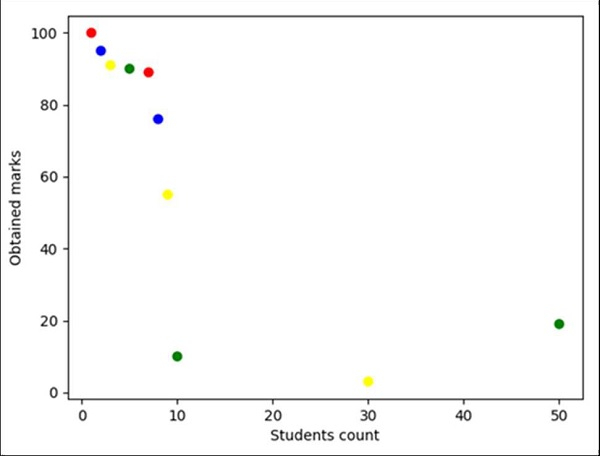# Plot different colors for different categorical levels using matplotlib

We can plot a diagram where a number of students will be plotted on the X-axis and the marks obtained by them will be plotted on the Y-axis. Also, we can set the color for different marks obtained by the students.

## Steps

• Make a list of the number of students.

• Make a list of marks that have been obtained by the students.

• To represent the color of each scattered point, we can have a list of colors.

• Using Panda, we can have a list representing the axes of the data frame.

• Create fig and ax variables using subplots method, where default nrows and ncols are 1.

• Set the “Students count” label using the plt.xlabel() method.

• Set the “Obtained marks” label using the plt.ylabel() method.

• To create a scatter point use a data frame, i.e., created in step 4, points are students_count, marks and color.

• To show the figure, use the plt.show() method.

## Example

from matplotlib import pyplot as plt
import pandas as pd

no_of_students = [1, 2, 3, 5, 7, 8, 9, 10, 30, 50]
marks_obtained_by_student = [100, 95, 91, 90, 89, 76, 55, 10, 3, 19]
color_coding = ['red', 'blue', 'yellow', 'green', 'red', 'blue', 'yellow', 'green', 'yellow', 'green']

df = pd.DataFrame(dict(students_count=no_of_students,
marks=marks_obtained_by_student, color=color_coding))

fig, ax = plt.subplots()

plt.xlabel('Students count')
plt.ylabel('Obtained marks')

ax.scatter(df['students_count'], df['marks'], c=df['color'])

plt.show()

## Output